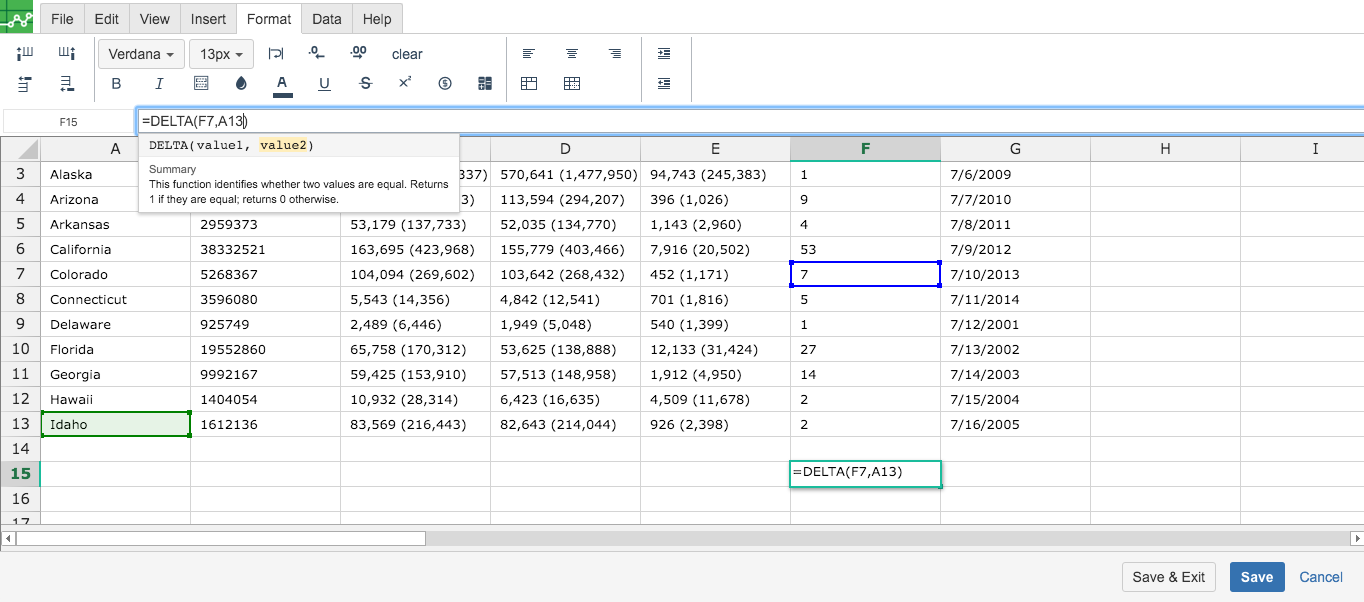# DELTA

### Definition of DELTA

Compare two numeric values, returning 1 if they're equal.

### Sample Usage

`DELTA(2, 1)`

`DELTA(A2)`

`DELTA(B3, 2)`

### Syntax

`DELTA(number1, [number2])`

• `number1` - the first number to compare.

• `number2` - [ OPTIONAL - `0` by default ] - the second number to compare.

### Notes

• If the second argument is not provided then `number1` will be compared to zero.
• A blank cell is treated the same as zero.
• This function only compares numbers. To compare other types of values, use the `EQ` function.

`EQ`: Returns "TRUE" if two specified values are equal and "FALSE" otherwise. Equivalent to the "=" operator.

`IF`: Returns one value if a logical expression is `TRUE` and another if it is `FALSE`.

`SUM`: Returns the sum of a series of numbers and/or cells.

### Once saved, your completed formula will display in a confluence page like this.

Error.

User does not have sufficient privileges to access this Content

##JavaScript errors detected